# Material parameter modeling

Sep. 26, 2010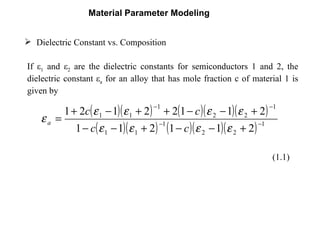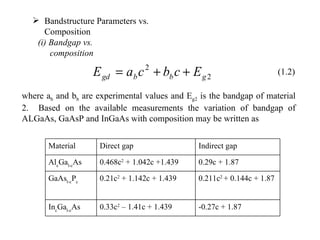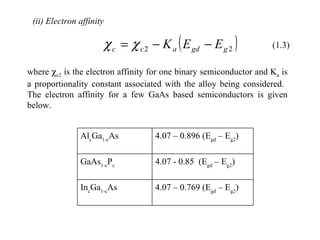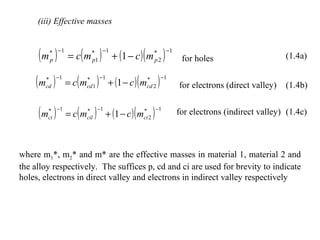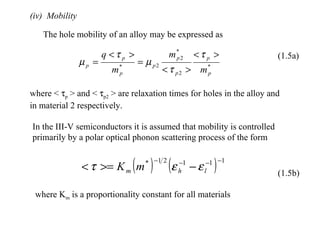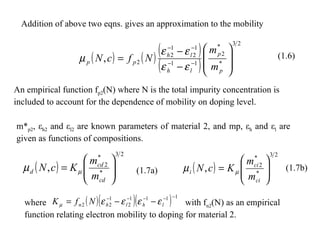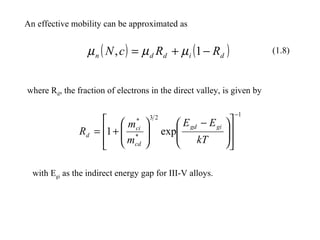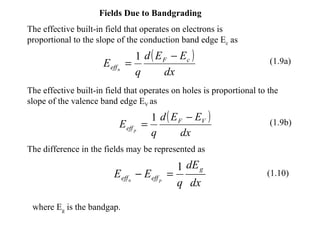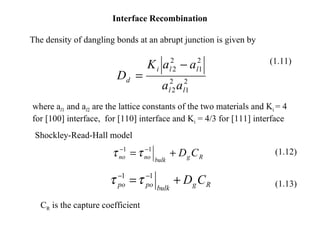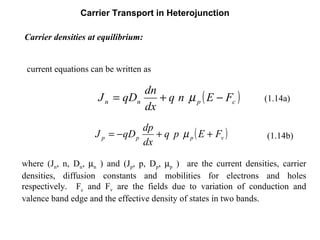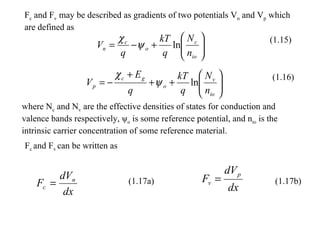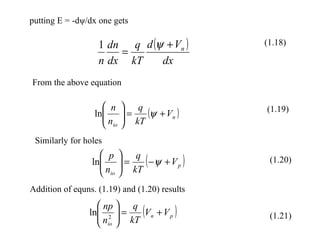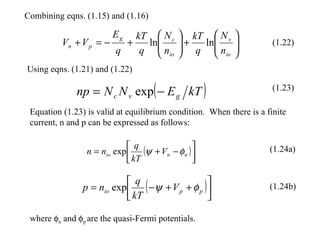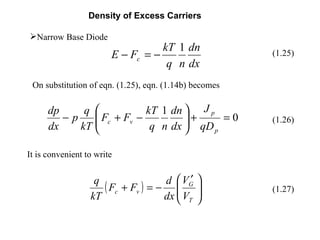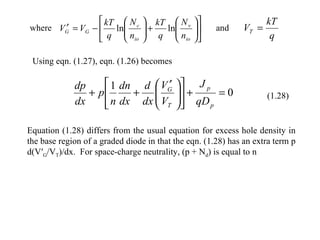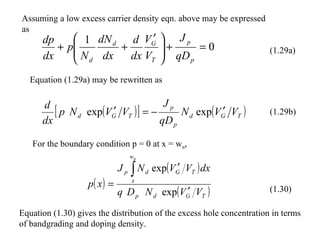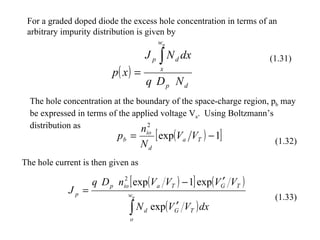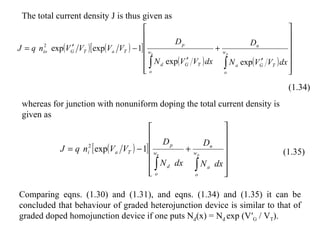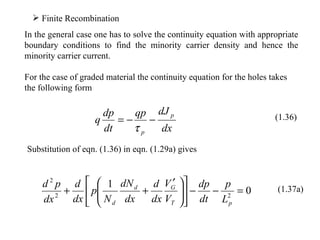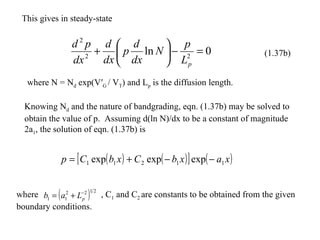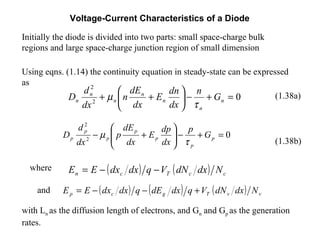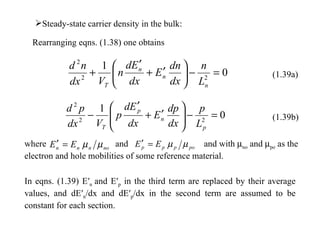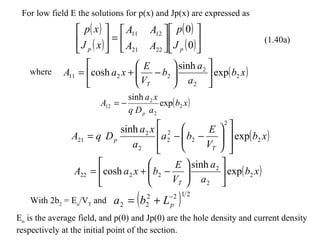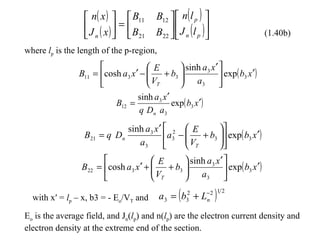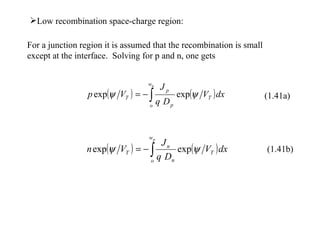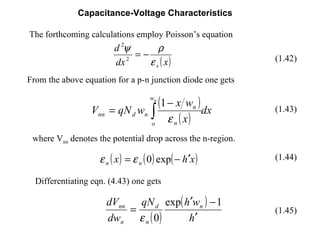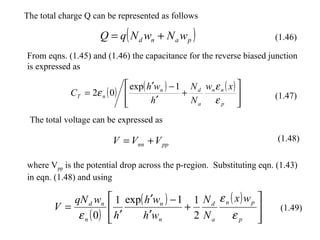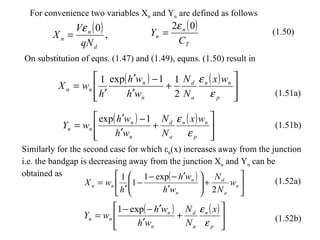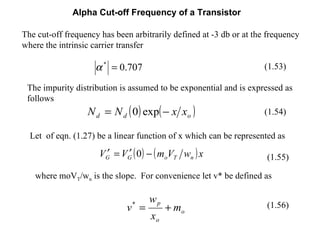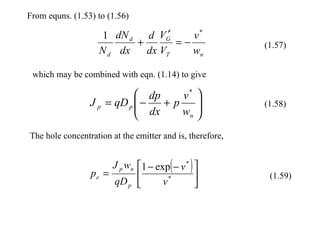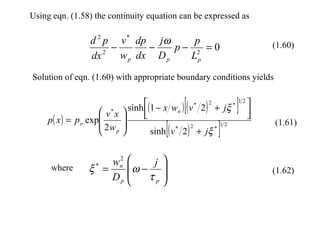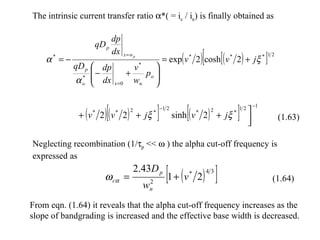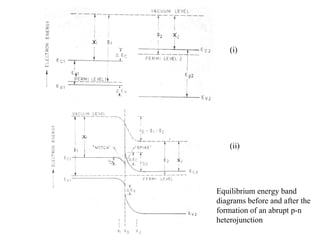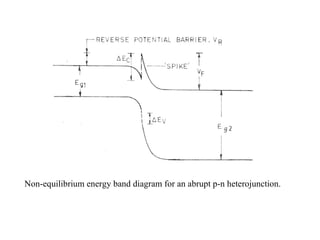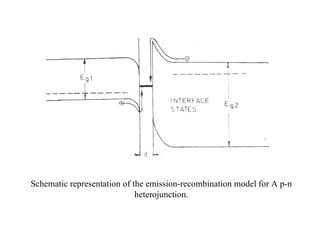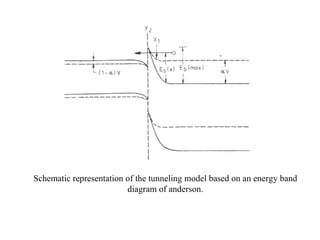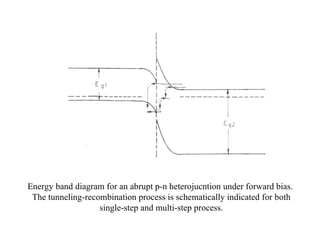1 of 37

### Material parameter modeling

• 1.
• 2.
• 3. (ii) Electron affinity where  c2 is the electron affinity for one binary semiconductor and K a is a proportionality constant associated with the alloy being considered. The electron affinity for a few GaAs based semiconductors is given below. (1.3) 4.07 – 0.769 (E gd – E g2 ) In c Ga 1-c As 4.07 - 0.85 (E gd – E g2 ) GaAs 1-c P c 4.07 – 0.896 (E gd – E g2 ) Al c Ga 1-c As
• 4. (iii) Effective masses for holes for electrons (direct valley) for electrons (indirect valley) where m 1 *, m 2 * and m* are the effective masses in material 1, material 2 and the alloy respectively. The suffices p, cd and ci are used for brevity to indicate holes, electrons in direct valley and electrons in indirect valley respectively (1.4a) (1.4b) (1.4c)
• 5. (iv) Mobility The hole mobility of an alloy may be expressed as where <  p > and <  p2 > are relaxation times for holes in the alloy and in material 2 respectively. In the III-V semiconductors it is assumed that mobility is controlled primarily by a polar optical phonon scattering process of the form where K m is a proportionality constant for all materials (1.5a) (1.5b)
• 6. Addition of above two eqns. gives an approximation to the mobility An empirical function f p2 (N) where N is the total impurity concentration is included to account for the dependence of mobility on doping level. m* p2 , ε h2 and ε l2 are known parameters of material 2, and mp, ε h and ε l are given as functions of compositions. where with f n2 (N) as an empirical function relating electron mobility to doping for material 2. (1.6) (1.7a) (1.7b)
• 7. An effective mobility can be approximated as where R d , the fraction of electrons in the direct valley, is given by with E gi as the indirect energy gap for III-V alloys. (1.8)
• 8. Fields Due to Bandgrading The effective built-in field that operates on electrons is proportional to the slope of the conduction band edge E c as The effective built-in field that operates on holes is proportional to the slope of the valence band edge E V as The difference in the fields may be represented as where E g is the bandgap. (1.9a) (1.9b) (1.10)
• 9. Interface Recombination The density of dangling bonds at an abrupt junction is given by where a l 1 and a l 2 are the lattice constants of the two materials and K i = 4 for  interface, for  interface and K i = 4/3 for  interface C R is the capture coefficient Shockley-Read-Hall model (1.12) (1.11) (1.13)
• 10. Carrier Transport in Heterojunction Carrier densities at equilibrium: current equations can be written as where (J n , n, D n ,  n ) and (J p , p, D p ,  p ) are the current densities, carrier densities, diffusion constants and mobilities for electrons and holes respectively. F c and F v are the fields due to variation of conduction and valence band edge and the effective density of states in two bands. (1.14a) (1.14b)
• 11. F c and F v may be described as gradients of two potentials V n and V p which are defined as where N c and N v are the effective densities of states for conduction and valence bands respectively, ψ o is some reference potential, and n io is the intrinsic carrier concentration of some reference material. F c and F v can be written as (1.15) (1.16) (1.17a) (1.17b)
• 12. putting E = -dψ/dx one gets From the above equation Similarly for holes (1.18) (1.19) (1.20) (1.21) Addition of equns. (1.19) and (1.20) results
• 13. where  n and  p are the quasi-Fermi potentials. Combining eqns. (1.15) and (1.16) Using eqns. (1.21) and (1.22) (1.22) Equation (1.23) is valid at equilibrium condition. When there is a finite current, n and p can be expressed as follows: (1.23) (1.24a) (1.24b)
• 14.
• 15. where and Using eqn. (1.27), eqn. (1.26) becomes (1.28) Equation (1.28) differs from the usual equation for excess hole density in the base region of a graded diode in that the eqn. (1.28) has an extra term p d(V′ G /V T )/dx. For space-charge neutrality, (p + N d ) is equal to n
• 16. For the boundary condition p = 0 at x = w n , Assuming a low excess carrier density eqn. above may be expressed as (1.29a) Equation (1.29a) may be rewritten as (1.29b) (1.30) Equation (1.30) gives the distribution of the excess hole concentration in terms of bandgrading and doping density.
• 17. For a graded doped diode the excess hole concentration in terms of an arbitrary impurity distribution is given by The hole concentration at the boundary of the space-charge region, p b may be expressed in terms of the applied voltage V a . Using Boltzmann’s distribution as (1.31) (1.32) The hole current is then given as (1.33)
• 18. The total current density J is thus given as whereas for junction with nonuniform doping the total current density is given as (1.34) Comparing eqns. (1.30) and (1.31), and eqns. (1.34) and (1.35) it can be concluded that behaviour of graded heterojunction device is similar to that of graded doped homojunction device if one puts N d (x) = N d exp (V′ G / V T ). (1.35)
• 19.
• 20. where , C 1 and C 2 are constants to be obtained from the given boundary conditions. This gives in steady-state (1.37b) where N = N d exp(V′ G / V T ) and L p is the diffusion length. Knowing N d and the nature of bandgrading, eqn. (1.37b) may be solved to obtain the value of p. Assuming d(ln N)/dx to be a constant of magnitude 2a 1 , the solution of eqn. (1.37b) is
• 21. Voltage-Current Characteristics of a Diode where and Initially the diode is divided into two parts: small space-charge bulk regions and large space-charge junction region of small dimension Using eqns. (1.14) the continuity equation in steady-state can be expressed as (1.38a) (1.38b) with L n as the diffusion length of electrons, and G n and G p as the generation rates.
• 22.
• 23. where For low field E the solutions for p(x) and Jp(x) are expressed as (1.40a) With 2b 2 = E o /V T and E o is the average field, and p(0) and Jp(0) are the hole density and current density respectively at the initial point of the section.
• 24. where l p is the length of the p-region, (1.40b) with x′ = l p – x, b3 = - E o /V T and E o is the average field, and J n ( l p ) and n( l p ) are the electron current density and electron density at the extreme end of the section.
• 25.
• 26. Capacitance-Voltage Characteristics The forthcoming calculations employ Poisson’s equation (1.42) From the above equation for a p-n junction diode one gets (1.43) where V nn denotes the potential drop across the n-region. (1.44) Differentiating eqn. (4.43) one gets (1.45)
• 27. The total charge Q can be represented as follows (1.46) From eqns. (1.45) and (1.46) the capacitance for the reverse biased junction is expressed as (1.47) The total voltage can be expressed as (1.48) where V pp is the potential drop across the p-region. Substituting eqn. (1.43) in eqn. (1.48) and using (1.49)
• 28. For convenience two variables X n and Y n are defined as follows (1.50) On substitution of eqns. (1.47) and (1.49), equns. (1.50) result in (1.51a) (1.51b) Similarly for the second case for which ε n (x) increases away from the junction i.e. the bandgap is decreasing away from the junction X n and Y n can be obtained as (1.52a) (1.52b)
• 29. Alpha Cut-off Frequency of a Transistor The cut-off frequency has been arbitrarily defined at -3 db or at the frequency where the intrinsic carrier transfer (1.53) The impurity distribution is assumed to be exponential and is expressed as follows (1.54) Let of eqn. (1.27) be a linear function of x which can be represented as (1.55) where moV T /w n is the slope. For convenience let v* be defined as (1.56)
• 30. From equns. (1.53) to (1.56) (1.57) which may be combined with eqn. (1.14) to give (1.58) The hole concentration at the emitter and is, therefore, (1.59)
• 31. Using eqn. (1.58) the continuity equation can be expressed as (1.60) Solution of eqn. (1.60) with appropriate boundary conditions yields (1.61) where (1.62)
• 32. The intrinsic current transfer ratio  *( = i c / i e ) is finally obtained as (1.63) Neglecting recombination (1/  p <<  ) the alpha cut-off frequency is expressed as (1.64) From eqn. (1.64) it reveals that the alpha cut-off frequency increases as the slope of bandgrading is increased and the effective base width is decreased.
• 33. Equilibrium energy band diagrams before and after the formation of an abrupt p-n heterojunction (i) (ii)
• 34. Non-equilibrium energy band diagram for an abrupt p-n heterojunction.
• 35. Schematic representation of the emission-recombination model for A p-n heterojunction.
• 36. Schematic representation of the tunneling model based on an energy band diagram of anderson.
• 37. Energy band diagram for an abrupt p-n heterojucntion under forward bias. The tunneling-recombination process is schematically indicated for both single-step and multi-step process.